Скачать презентацию Approximating Node-Weighted Survivable Networks Zeev Nutov The Open

4a8ea0f26aa082d3c273dde9e90d9e01.ppt

• Количество слайдов: 23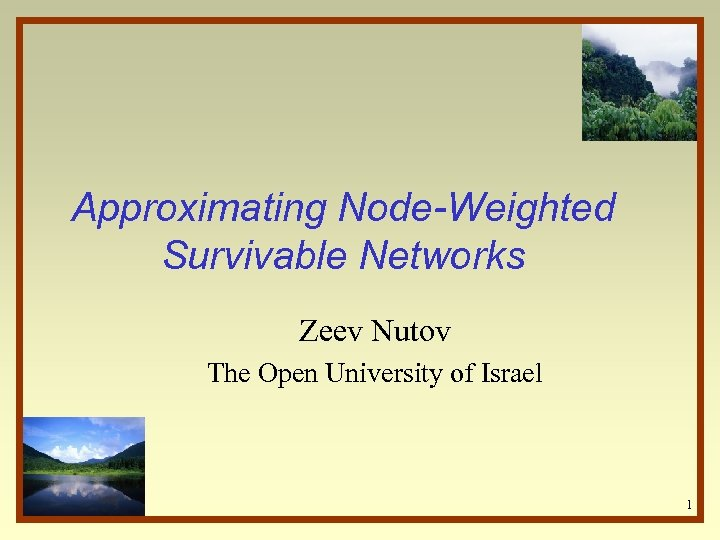Approximating Node-Weighted Survivable Networks Zeev Nutov The Open University of Israel 1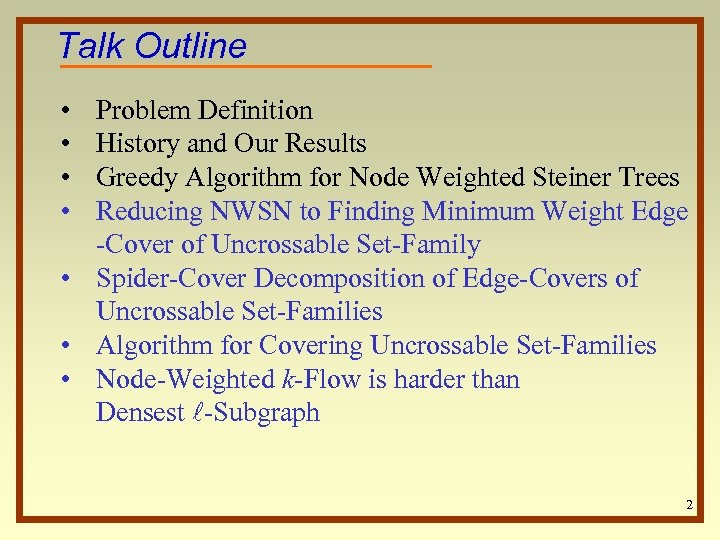Talk Outline • • Problem Definition History and Our Results Greedy Algorithm for Node Weighted Steiner Trees Reducing NWSN to Finding Minimum Weight Edge -Cover of Uncrossable Set-Family • Spider-Cover Decomposition of Edge-Covers of Uncrossable Set-Families • Algorithm for Covering Uncrossable Set-Families • Node-Weighted k-Flow is harder than Densest l-Subgraph 2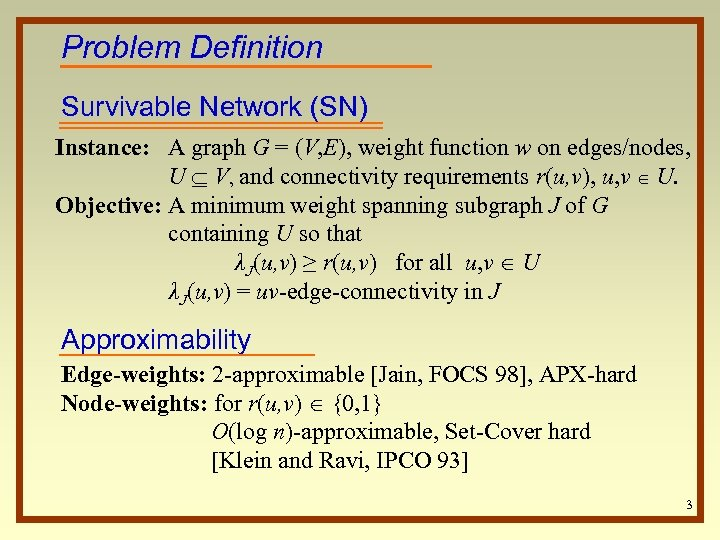Problem Definition Survivable Network (SN) Instance: A graph G = (V, E), weight function w on edges/nodes, U V, and connectivity requirements r(u, v), u, v U. Objective: A minimum weight spanning subgraph J of G containing U so that λJ(u, v) ≥ r(u, v) for all u, v U λJ(u, v) = uv-edge-connectivity in J Approximability Edge-weights: 2 -approximable [Jain, FOCS 98], APX-hard Node-weights: for r(u, v) {0, 1} O(log n)-approximable, Set-Cover hard [Klein and Ravi, IPCO 93] 3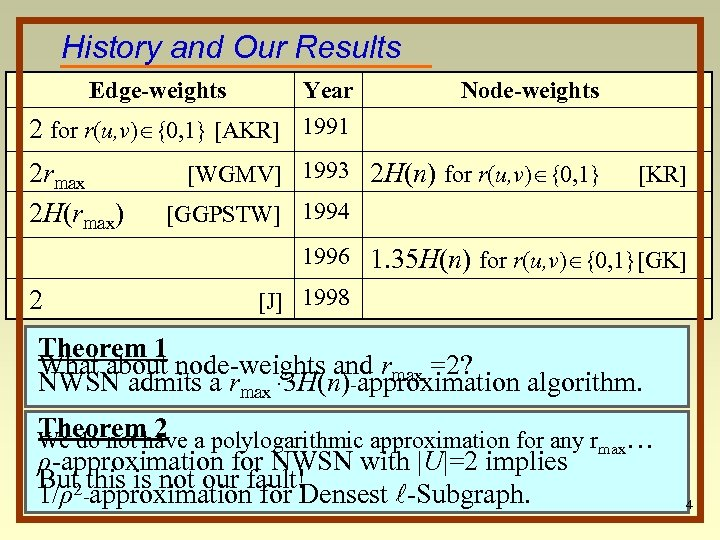History and Our Results Edge-weights Year 2 for r(u, v) {0, 1} [AKR] 1991 2 rmax 2 H(rmax) Node-weights [WGMV] 1993 2 H(n) for r(u, v) {0, 1} [KR] [GGPSTW] 1994 1996 1. 35 H(n) for r(u, v) {0, 1}[GK] 2 [J] 1998 Theorem 1 What about node-weights and rmax =2? NWSN admits a rmax · 3 H(n)-approximation algorithm. Theorem 2 We do not have a polylogarithmic approximation for any rmax… ρ-approximation for NWSN with |U|=2 implies But 2 this is not our fault! 1/ρ -approximation for Densest l-Subgraph. 4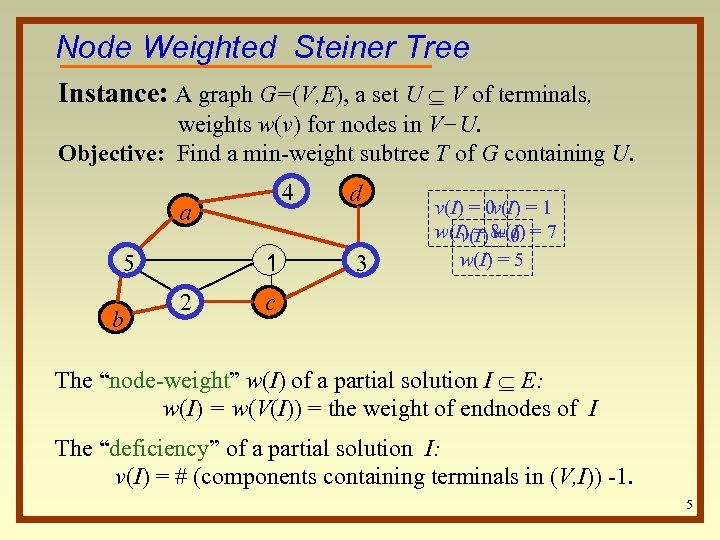Node Weighted Steiner Tree Instance: A graph G=(V, E), a set U V of terminals, weights w(v) for nodes in V−U. Objective: Find a min-weight subtree T of G containing U. 4 a 1 5 b 2 d 3 v(I) = 0 v(I) = 1 w(I) = 8 0 = 7 v(I) w(I) = 5 c The “node-weight” w(I) of a partial solution I E: w(I) = w(V(I)) = the weight of endnodes of I The “deficiency” of a partial solution I: v(I) = # (components containing terminals in (V, I)) -1. 5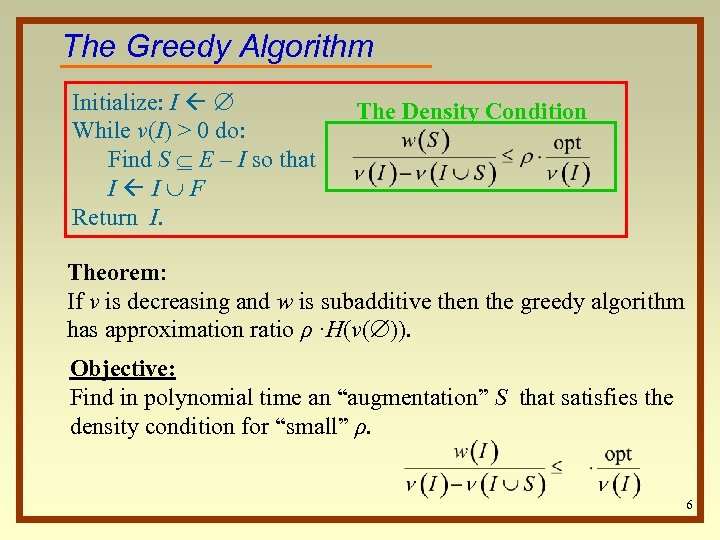The Greedy Algorithm Initialize: I While ν(I) > 0 do: Find S E – I so that I I F Return I. The Density Condition Theorem: If ν is decreasing and w is subadditive then the greedy algorithm has approximation ratio ρ ·H(ν( )). Objective: Find in polynomial time an “augmentation” S that satisfies the density condition for “small” ρ. 6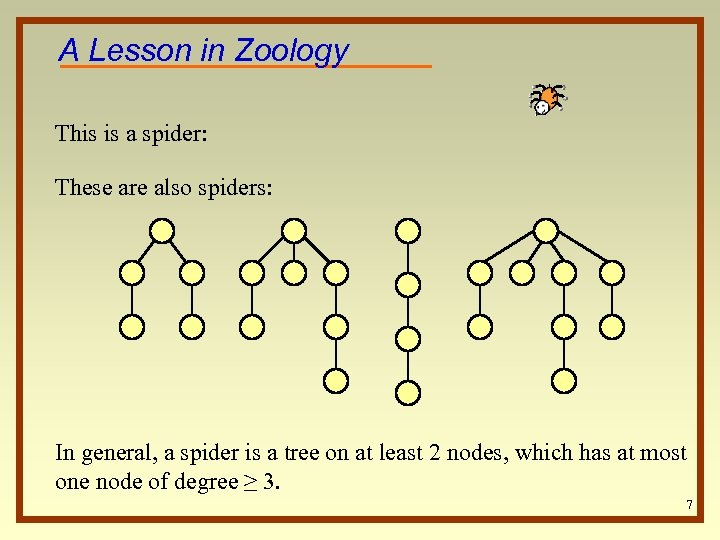A Lesson in Zoology This is a spider: These are also spiders: In general, a spider is a tree on at least 2 nodes, which has at most one node of degree ≥ 3. 7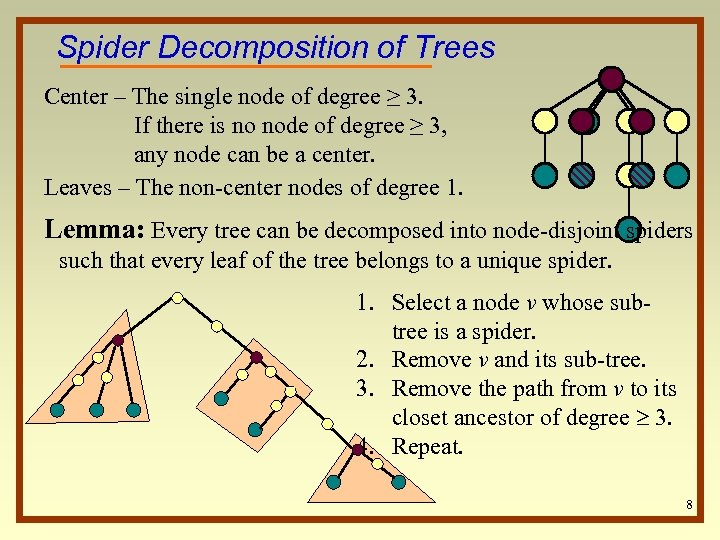Spider Decomposition of Trees Center – The single node of degree ≥ 3. If there is no node of degree ≥ 3, any node can be a center. Leaves – The non-center nodes of degree 1. Lemma: Every tree can be decomposed into node-disjoint spiders such that every leaf of the tree belongs to a unique spider. 1. Select a node v whose subtree is a spider. 2. Remove v and its sub-tree. 3. Remove the path from v to its closet ancestor of degree 3. 4. Repeat. 8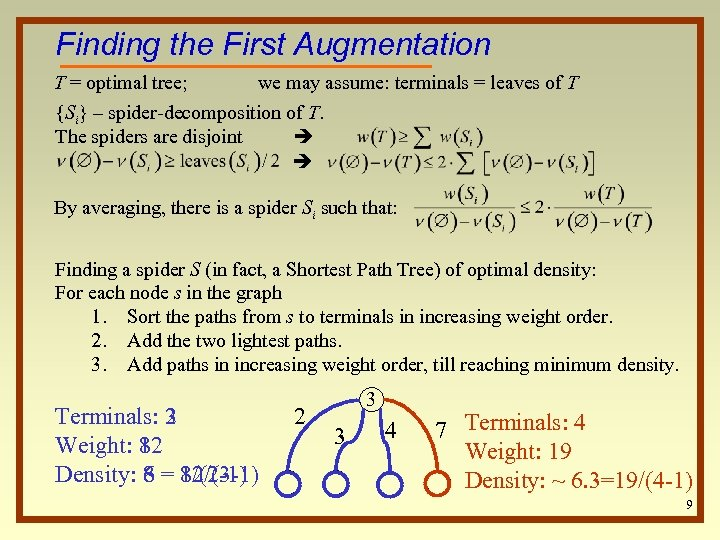Finding the First Augmentation T = optimal tree; we may assume: terminals = leaves of T {Si} – spider-decomposition of T. The spiders are disjoint By averaging, there is a spider Si such that: Finding a spider S (in fact, a Shortest Path Tree) of optimal density: For each node s in the graph 1. Sort the paths from s to terminals in increasing weight order. 2. Add the two lightest paths. 3. Add paths in increasing weight order, till reaching minimum density. Terminals: 2 3 Weight: 8 12 Density: 8 = 8/(2 -1) 6 12/(3 -1) 2 3 3 4 7 Terminals: 4 Weight: 19 Density: ~ 6. 3=19/(4 -1) 9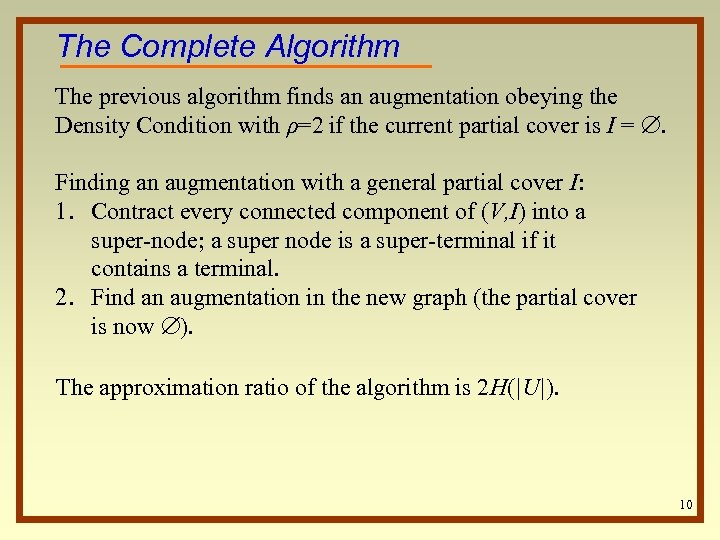The Complete Algorithm The previous algorithm finds an augmentation obeying the Density Condition with ρ=2 if the current partial cover is I = . Finding an augmentation with a general partial cover I: 1. Contract every connected component of (V, I) into a super-node; a super node is a super-terminal if it contains a terminal. 2. Find an augmentation in the new graph (the partial cover is now ). The approximation ratio of the algorithm is 2 H(|U|). 10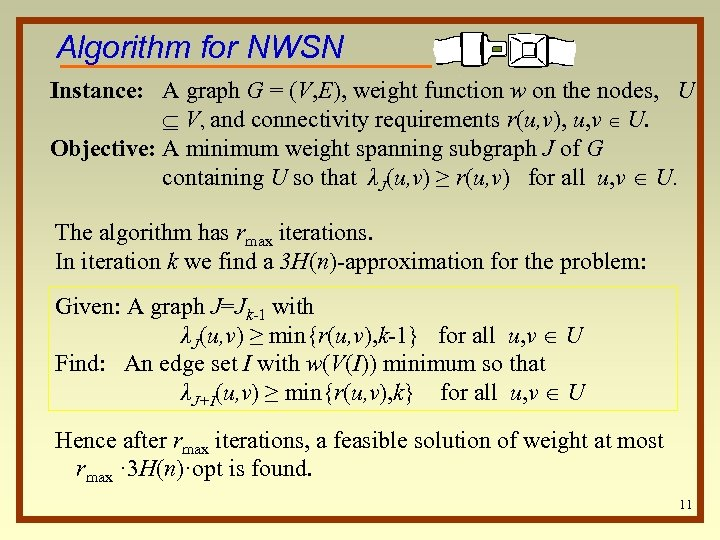Algorithm for NWSN Instance: A graph G = (V, E), weight function w on the nodes, U V, and connectivity requirements r(u, v), u, v U. Objective: A minimum weight spanning subgraph J of G containing U so that λJ(u, v) ≥ r(u, v) for all u, v U. The algorithm has rmax iterations. In iteration k we find a 3 H(n)-approximation for the problem: Given: A graph J=Jk-1 with λJ(u, v) ≥ min{r(u, v), k-1} for all u, v U Find: An edge set I with w(V(I)) minimum so that λJ+I(u, v) ≥ min{r(u, v), k} for all u, v U Hence after rmax iterations, a feasible solution of weight at most rmax · 3 H(n)·opt is found. 11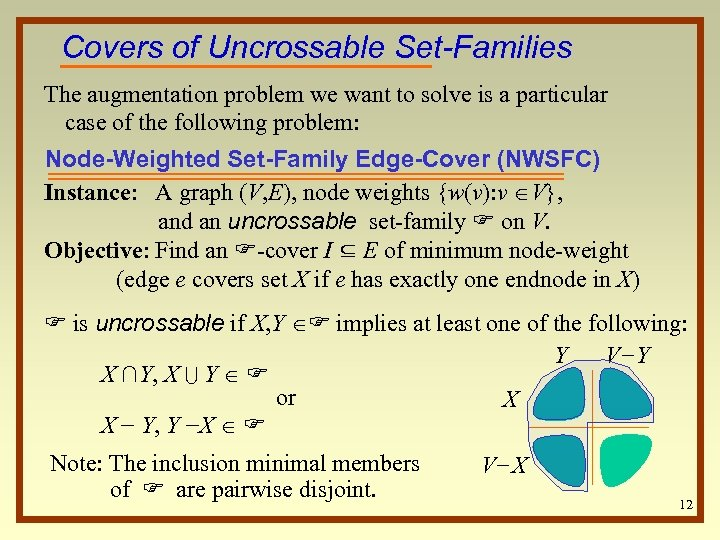Covers of Uncrossable Set-Families The augmentation problem we want to solve is a particular case of the following problem: Node-Weighted Set-Family Edge-Cover (NWSFC) Instance: A graph (V, E), node weights {w(v): v V}, and an uncrossable set-family F on V. Objective: Find an F-cover I ⊆ E of minimum node-weight (edge e covers set X if e has exactly one endnode in X) F is uncrossable if X, Y F implies at least one of the following: Y V−Y X ∩Y, X U Y F or X X − Y, Y −X F Note: The inclusion minimal members of F are pairwise disjoint. V−X 12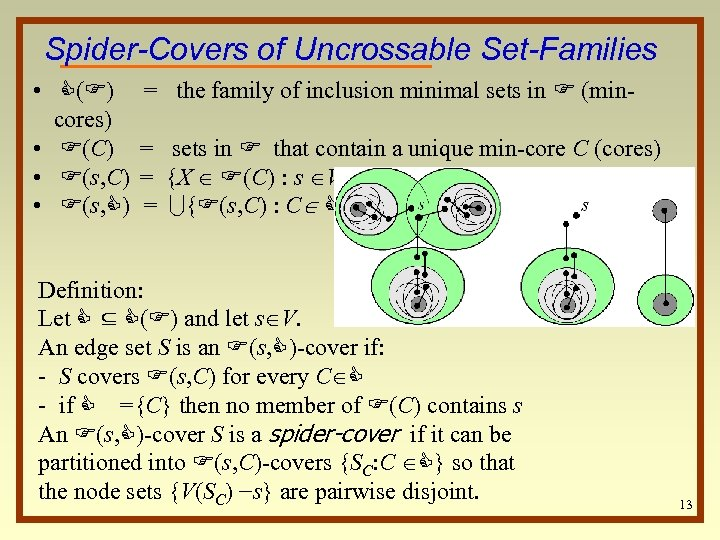Spider-Covers of Uncrossable Set-Families • C(F) cores) • F(C) • F(s, C) = the family of inclusion minimal sets in F (min= sets in F that contain a unique min-core C (cores) = {X F(C) : s V-X} = U{F(s, C) : C C} Definition: Let C ⊆ C(F) and let s V. An edge set S is an F(s, C)-cover if: - S covers F(s, C) for every C C - if C ={C} then no member of F(C) contains s An F(s, C)-cover S is a spider-cover if it can be partitioned into F(s, C)-covers {SC: C C} so that the node sets {V(SC) −s} are pairwise disjoint. 13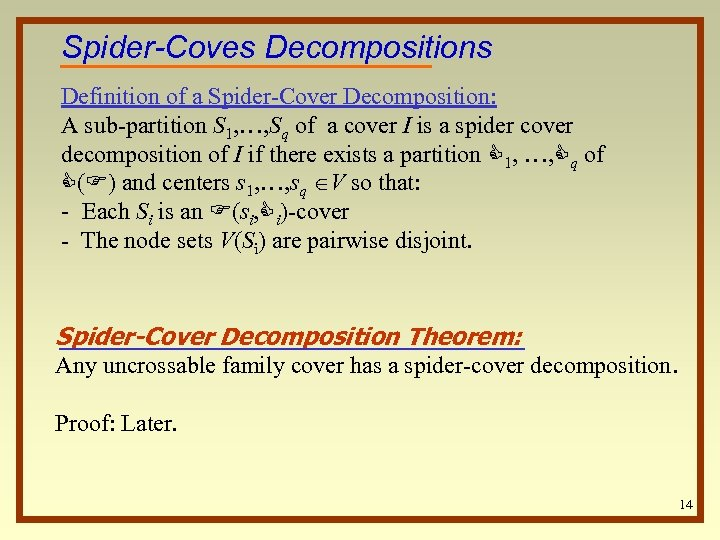Spider-Coves Decompositions Definition of a Spider-Cover Decomposition: A sub-partition S 1, …, Sq of a cover I is a spider cover decomposition of I if there exists a partition C 1, …, Cq of C(F) and centers s 1, …, sq V so that: - Each Si is an F(si, Ci)-cover - The node sets V(Si) are pairwise disjoint. Spider-Cover Decomposition Theorem: Any uncrossable family cover has a spider-cover decomposition. Proof: Later. 14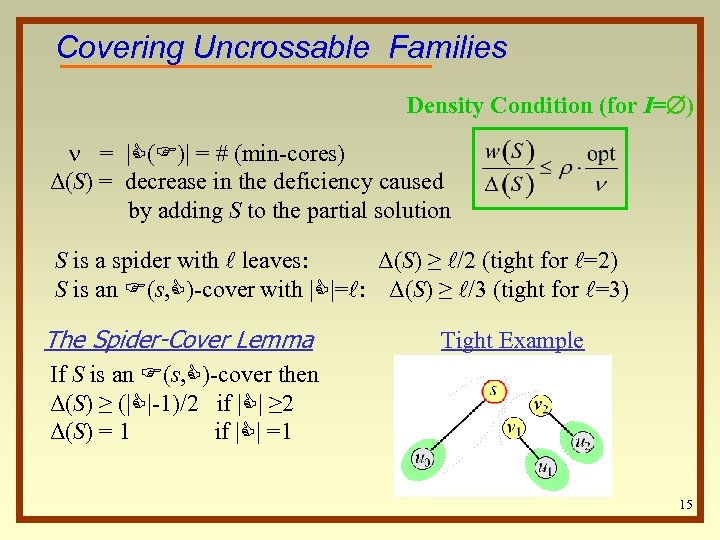Covering Uncrossable Families Density Condition (for I= ) = |C(F)| = # (min-cores) Δ(S) = decrease in the deficiency caused by adding S to the partial solution S is a spider with l leaves: Δ(S) ≥ l/2 (tight for l=2) S is an F(s, C)-cover with |C|=l: Δ(S) ≥ l/3 (tight for l=3) The Spider-Cover Lemma Tight Example If S is an F(s, C)-cover then Δ(S) ≥ (|C|-1)/2 if |C| ≥ 2 Δ(S) = 1 if |C| =1 15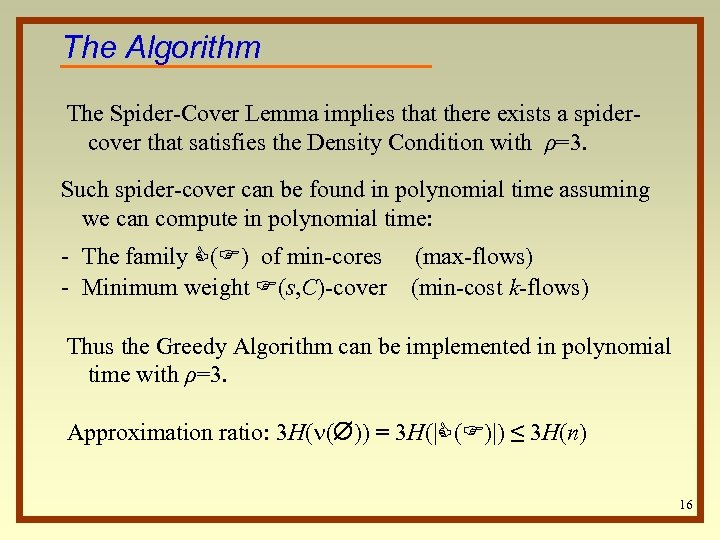The Algorithm The Spider-Cover Lemma implies that there exists a spidercover that satisfies the Density Condition with ρ=3. Such spider-cover can be found in polynomial time assuming we can compute in polynomial time: - The family C(F) of min-cores (max-flows) - Minimum weight F(s, C)-cover (min-cost k-flows) Thus the Greedy Algorithm can be implemented in polynomial time with ρ=3. Approximation ratio: 3 H( ( )) = 3 H(|C(F)|) ≤ 3 H(n) 16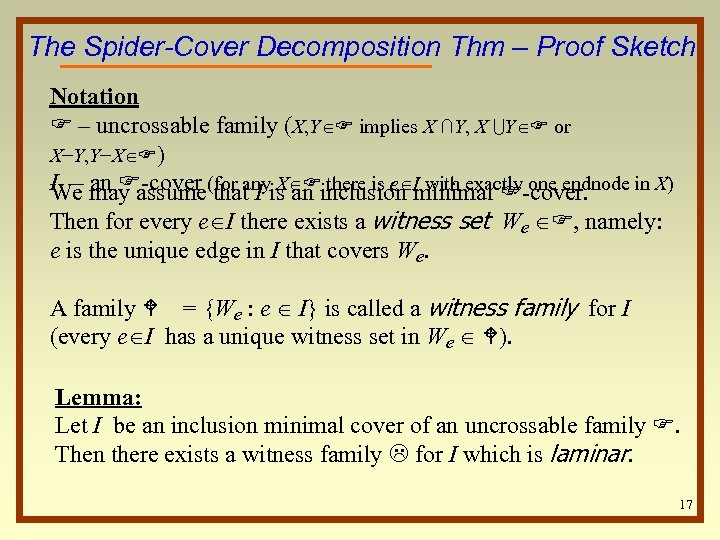The Spider-Cover Decomposition Thm – Proof Sketch Notation F – uncrossable family (X, Y F implies X ∩Y, X UY F or X−Y, Y−X F) I – may assume(for anyis an inclusion minimal F-cover. We an F-cover that I X F there is e I with exactly one endnode in X) Then for every e I there exists a witness set We F, namely: e is the unique edge in I that covers We. A family W = {We : e I} is called a witness family for I (every e I has a unique witness set in We W). Lemma: Let I be an inclusion minimal cover of an uncrossable family F. Then there exists a witness family L for I which is laminar. 17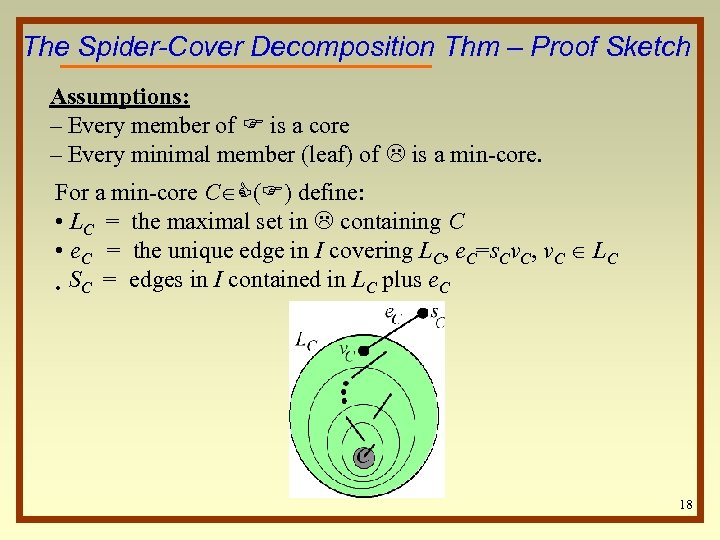The Spider-Cover Decomposition Thm – Proof Sketch Assumptions: – Every member of F is a core – Every minimal member (leaf) of L is a min-core. For a min-core C C(F) define: • LC = the maximal set in L containing C • e. C = the unique edge in I covering LC, e. C=s. Cv. C, v. C LC • SC = edges in I contained in LC plus e. C 18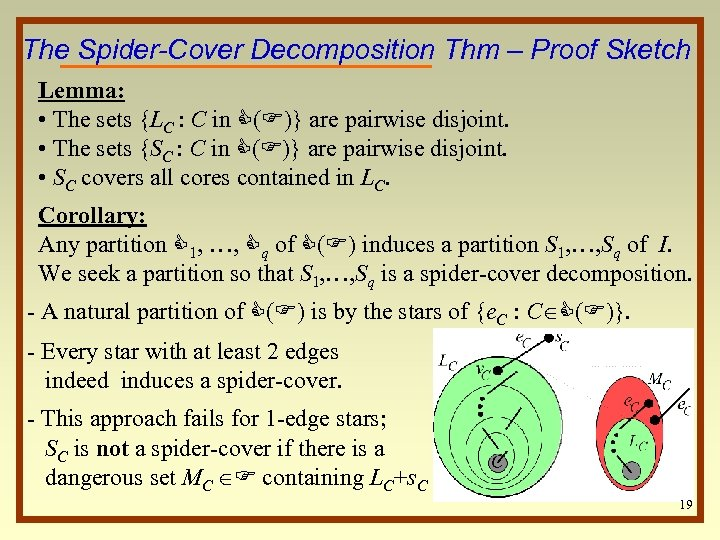The Spider-Cover Decomposition Thm – Proof Sketch Lemma: • The sets {LC : C in C(F)} are pairwise disjoint. • The sets {SC : C in C(F)} are pairwise disjoint. • SC covers all cores contained in LC. Corollary: Any partition C 1, …, Cq of C(F) induces a partition S 1, …, Sq of I. We seek a partition so that S 1, …, Sq is a spider-cover decomposition. - A natural partition of C(F) is by the stars of {e. C : C C(F)}. - Every star with at least 2 edges indeed induces a spider-cover. - This approach fails for 1 -edge stars; SC is not a spider-cover if there is a dangerous set MC F containing LC+s. C 19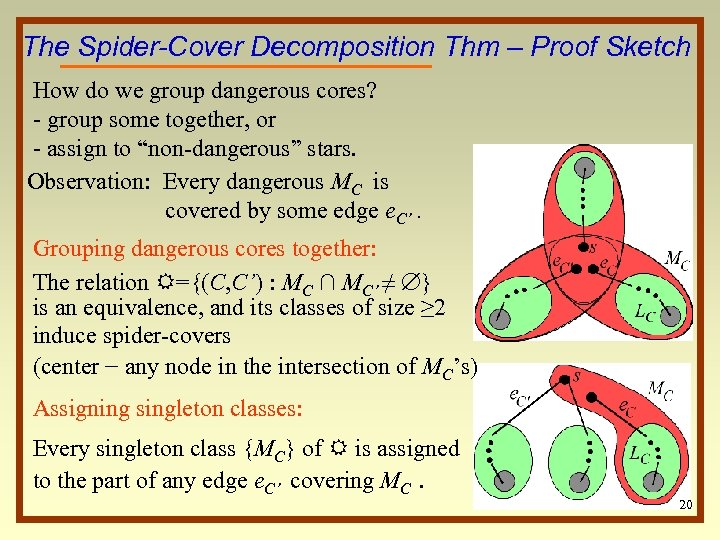The Spider-Cover Decomposition Thm – Proof Sketch How do we group dangerous cores? - group some together, or - assign to “non-dangerous” stars. Observation: Every dangerous MC is covered by some edge e. C’. Grouping dangerous cores together: The relation R={(C, C’) : MC ∩ MC’ ≠ } is an equivalence, and its classes of size ≥ 2 induce spider-covers (center − any node in the intersection of MC’s) Assigning singleton classes: Every singleton class {MC} of R is assigned to the part of any edge e. C’ covering MC. 20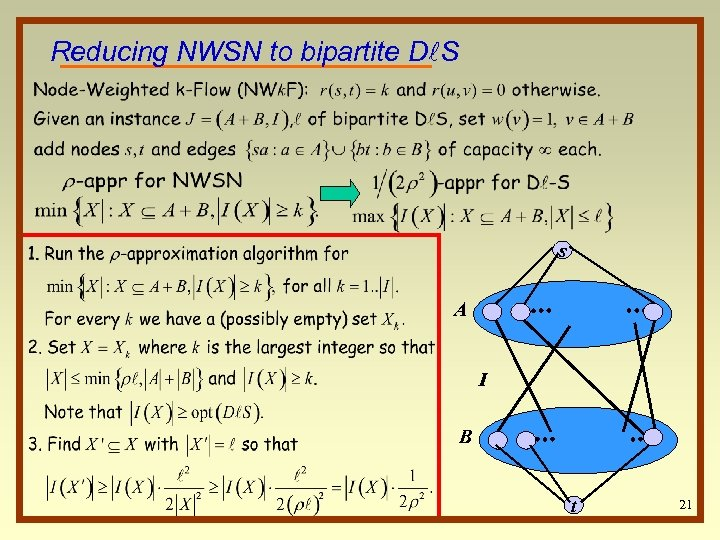Reducing NWSN to bipartite Dl. S s A I B t 21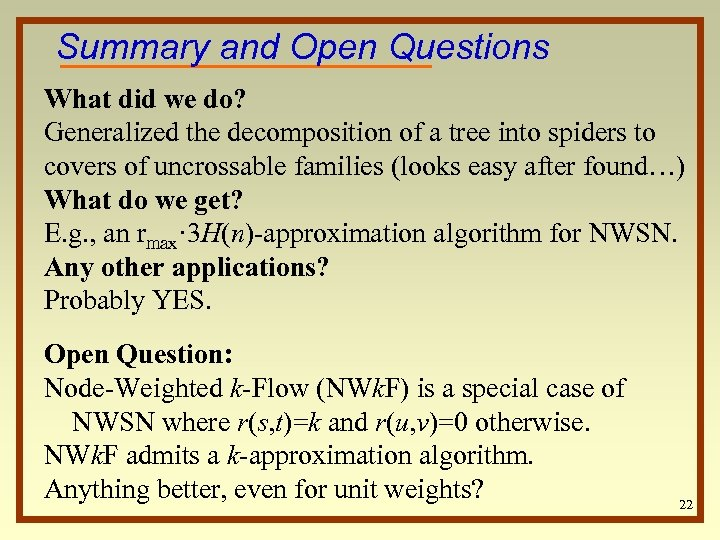Summary and Open Questions What did we do? Generalized the decomposition of a tree into spiders to covers of uncrossable families (looks easy after found…) What do we get? E. g. , an rmax· 3 H(n)-approximation algorithm for NWSN. Any other applications? Probably YES. Open Question: Node-Weighted k-Flow (NWk. F) is a special case of NWSN where r(s, t)=k and r(u, v)=0 otherwise. NWk. F admits a k-approximation algorithm. Anything better, even for unit weights? 2223